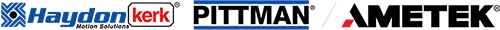# Stepper Motors the Effect of Acceleration Ramps

This white paper explains stand test methodology for generating force versus velocity curves and the benefits of adding acceleration and deceleration ramps.

One of the first parameters that is considered when choosing a stepper motor based linear actuator for an application is how much force can the actuator produce. So naturally a speed versus force curve is the first place someone looks. There are a couple of different ways to present these speed vs. force curves, one is force versus pulse rate and the second is force versus linear velocity. The maximum force at various step rates throughout the usable speed range for each type of actuator is recorded and used to generate the curves. The most difficult load condition is when a vertical load is fully supported by the actuator at all times. To test under these conditions a custom gantry was created to hold the actuator with proper mechanical alignment, apply the load and also provide a means of measuring the displacement of the actuator, all at the same time. Figure is a picture of the gantry with a size 17 single stack actuator.

A stepper motor drive is the other important piece of equipment that is necessary to perform this experiment. With the purpose of this experiment being to show the benefits of adding acceleration to a run profile, a chopper drive had to be used. A PCM4826 along with the IDEA drive software was used to drive the motor. A 40 volt power supply was used to power the drive along with the use of a 5 volt motor coil to maintain the 8 to 1 voltage ratio.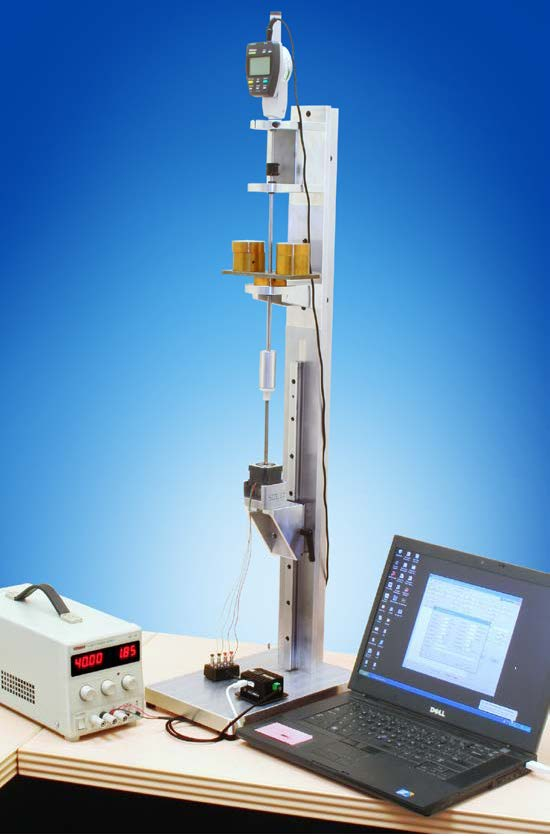Figure 1:Computer programmable test system.

The 8 to 1 voltage ratio was used so the optimum performance of the motor could be achieved. At this point a simple run program was created to have the motor extend, pause, and then retract. In the extend command, the inputs to the run profile were as follows, Figure 2:

 Distance: 800 steps Speed: 1600 steps/sec Run Current: 0.7 Arms (full rated current) Hold Current: 0.4 Arms Delay Tim: 0.05 sec Step Mode: 1 (full step mode) Accel Rate: 0 steps/sec/sec Decel Rate: 0 steps/sec/sec Start Speed: 0 steps/sec End Speed: 0 steps/sec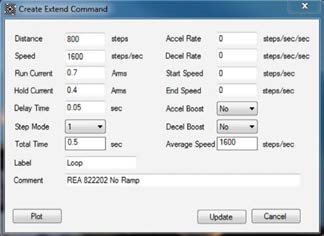Figure 2: Screen image of “Extend Command” program.

When this move is plotted you can see Figure 3, that the drive immediately requests the motor to start moving at 1600 full steps per second because there is no acceleration used. The motor has to overcome rotor inertia and the dead weight that is on the gantry instantaneously.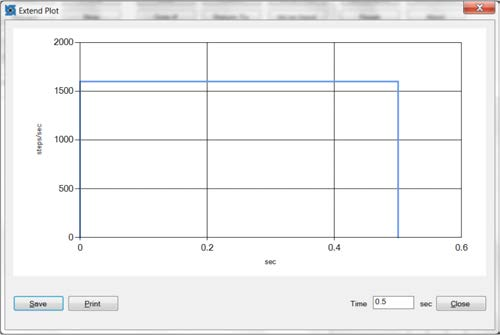Figure 3: Screen image of move profile.

This move profile, Figure 4, was put into a loop so it would repeat until manually stopped. This made the actuator cycle up and down at the designated speed. While the actuator moved the dead weight loading was incrementally increased until the maximum operational output force was obtained just at the point of stalling.
The indicator at the top of the gantry provided feedback as to whether or not the actuator experienced stalling or missed steps and the weight was recorded.Figure 4: Screen image of program set to loop motion.

The speed was the only parameter that was changed in order to generate a speed versus force curve. In this experiment speeds from 100 to 2500 full steps per second were tested. After all the data points were taken without using an acceleration profile the extend command needed to be adjusted to include the acceleration
ramp. The inputs to the run profile in the extend command with acceleration were as follows Figure 5:

 Distance: 800 steps Speed: 1600 steps/sec Run Current: 0.7 Arms (full rated current) Hold Current: 0.4 Arms Delay Tim: 0.05 sec Step Mode: 1 (full step mode) Accel Rate: 5000 steps/sec/sec Decel Rate: 50000 steps/sec/sec Start Speed: 400 steps/sec End Speed: 400 steps/sec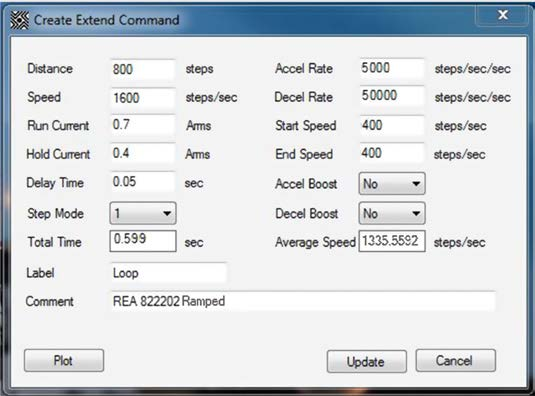Figure 5: Screen image of program with acceleration ramp.

When this move is plotted you can see Figure 6, that the drive requests the motor to start moving at 400 full steps per second and then accelerate up to 1600 full steps per second at a rate of 5000 full steps per second squared. The speed is then held constant until it is time to slow down where a steep deceleration of 50000 full steps per second squared was used until a speed of 400 full steps per second and then the motor was commanded to stop. With acceleration added to the move, the motor still has to overcome rotor inertia along with the weight on the gantry but at a slower initial speed and then increased until top speed is achieved.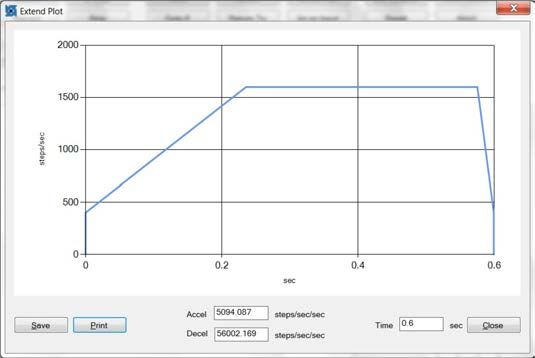Figure 6: Acceleration, constant speed and deceleration.

Again, data points were taken while varying the speed from 100 to 2500 full steps per second and the maximum weight before missed steps or stall occurred was recorded. Below in Figure 7, you can see the force versus speed (pulse rate) plot with and without acceleration.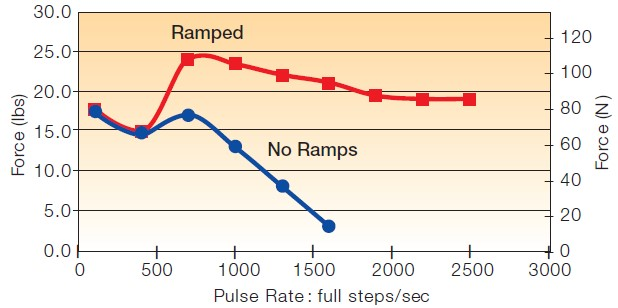Figure 7: Test results: Force versus Speed (Pulse Rate)

The use of an acceleration ramp greatly affects the performance of a stepper motor. In this experiment the actuator was in a vertical orientation so the use of an acceleration ramp allowed the motor to move much more weight. This is because the motor was asked to start moving at a slower speed and then gradually increase its
speed until the top speed was achieved. A very steep deceleration was used to help slow down the load before the actuator was commanded to stop. During an extend move in a vertical application the weight naturally wants to slow down so the use of a long deceleration is not necessary. Acceleration ramps really only have
benefits when using an actuator at high speeds which can be seen in the plot above. The dip in the plot is caused by resonance. This is when the step rate equals the natural frequency of the motor. Acceleration and deceleration ramps can help the motor get through this resonance zone as quickly as possible to reduce the
effects.

The use of acceleration ramps is very application dependent. There are several things to take into consideration when determining a move profile some of these include:

 The available stroke of the actuator Type of load: frictional, inertial, mechanical spring, or a combination of these Orientation of load: horizontal, vertical, or in between Speed required Type of drive: L/R of chopper (can it accommodate acceleration/deceleration?) Drive current supplied The source voltage to motor voltage ratio
The stroke of the actuator will affect the acceleration as you will only have so much time to perform your complete desired move. The longer the stroke, the more total time you have for a move, so a longer acceleration could be used. The orientation of the load also influences the use of acceleration and deceleration profiles. For a horizontal movement both an acceleration and deceleration will be beneficial to get the load moving and then to help slow it down so the final position is not over shot.

Let’s take a look at the benefits of an acceleration ramp in a potential real world application. If a person was trying to size a motor for an application and needed to move a load of 15 lbs at a speed of 1.5” per second, one could conclude that the motor tested in this experiment would not be the correct choice when looking at
the curve with no ramping. In fact, this motor would work if the application allowed for an acceleration ramp. The motor tested in this experiment with an acceleration ramp was able to move a load of about 22 lbs which still provides some safety margin as well.

The plot in Figure 8 shows the force versus speed curves where these data points were taken. The actuator in this experiment had a linear travel of 0.00125 inches per step so the data points were converted from steps per second multiplied by the distance per step to get linear velocity of inches per second. It has been
learned empirically that a factor of 1.6 can be applied to a force curve without ramping to get an estimate of the potential force the actuator can output if an acceleration ramp is used.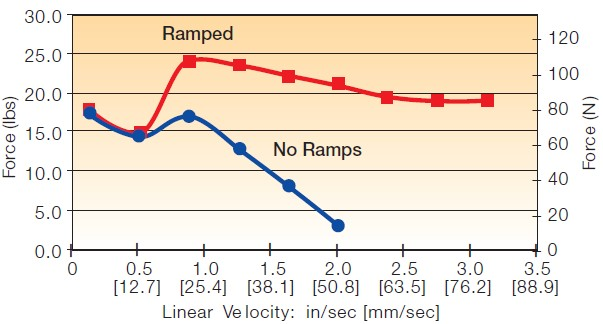Figure 8: Test results: Force versus Linear Velocity.

When evaluating a system’s needs and comparing them to the force output of a linear actuator it is important to remember that there are several factors that can improve or compromise the actuators performance. Some factors such as run current and voltage ratio are important factors. The addition of an acceleration and deceleration ramp to a move profile can be the difference in staying with the same size motor as appose to having to go with a larger, more powerful actuator. This experiment was designed to show the effects of using an acceleration ramp to allow the linear actuator to achieve higher loads and linear velocity than would be realized by following the standard performance curves.